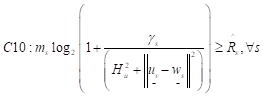# CVX rel_entr use for different types of formulas

how can i write this formula in cvx?

``````a*log2(1+b/c)
plz help me, as i write it as
a*log2(1+b/c)=-rel_entr(c,(c+b))/log(2);
``````

is it right or not?
plz suggest me.

it results ok in one condition but appears
Illegal operation: rel_entr( {convex}, {convex} )
in next condition for other form.

If more than one of a, b, c is a CVX variable, that is neither convex nor concave, and so can’t be used in CVX.

1 Like

i want to write this formula in cvx, plz suggest me how to write it correctly.
`R=x*log(2)(1+gama/(H^2+llu-wll^2))`
plz suggest me the right way to write it in cvx

gama=p*constant/x
x, p is variable,
u is the uav position vch s being changed
w is user

Please write a clear formula, showing explicitly what the optimization variables are, and what the input data is. Per you description, `x*log(2)(1+gama/(H^2+llu-wll^2))` has only the variable `x`, and the variable `p` does not even appear.ms is bandwidth
gama= depends on power and bandwidth
H is height
u and w are locations of UAV and user.
u is decision variable in this problem

I have no idea what any of that is. I have no idea what the variables are. Have you proven that it is jointly convex or concave with respect to the optimization variables?

it is nonconvex and i have to extract a value from it for comparison
i am trying since many days but could not reach any result

i am a student, working on thesis research work for my doctoral degree

If it is neither convex, nor concave, it can’t be used in CVX. If you really need to optimize with such expressions, use a non-convex solver, such as available under YALMIP.

i have the only problem with above equation
if i use -rel_entr(x,y) then ti shows
this error
Error using cvx/rel_entr (line 71)
Disciplined convex programming error:
Illegal operation: rel_entr( {convex}, {convex} ).

and when i use the above formula directly then it shows
this error
Error using .* (line 173)
Disciplined convex programming error:
Cannot perform the operation: {positive constant} ./ {convex}

Error in ./ (line 19)
z = times( x, y, ‘./’ );

presently i am not approaching my target
plz suggest me the better way for solution